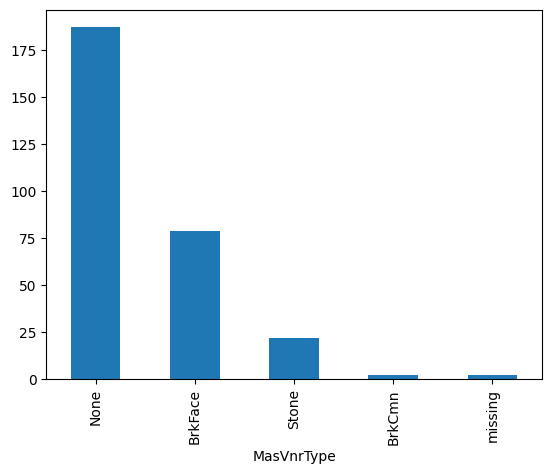# CategoricalImputer#

The `CategoricalImputer()` replaces missing data in categorical variables with an arbitrary value, like the string ‘Missing’ or by the most frequent category.

You can indicate which variables to impute passing the variable names in a list, or the imputer automatically finds and selects all variables of type object and categorical.

Originally, we designed this imputer to work only with categorical variables. From version 1.1.0 we introduced the parameter `ignore_format` to allow the imputer to also impute numerical variables with this functionality. This is, because in some cases, variables that are by nature categorical, have numerical values.

Below a code example using the House Prices Dataset (more details about the dataset here).

In this example, we impute 2 variables from the dataset with the string ‘Missing’, which is the default functionality of the transformer:

```import numpy as np
import pandas as pd
import matplotlib.pyplot as plt
from sklearn.model_selection import train_test_split

from feature_engine.imputation import CategoricalImputer

# Separate into train and test sets
X_train, X_test, y_train, y_test = train_test_split(
data.drop(['Id', 'SalePrice'], axis=1), data['SalePrice'], test_size=0.3, random_state=0)

# set up the imputer
imputer = CategoricalImputer(variables=['Alley', 'MasVnrType'])

# fit the imputer
imputer.fit(X_train)

# transform the data
train_t= imputer.transform(X_train)
test_t= imputer.transform(X_test)

test_t['MasVnrType'].value_counts().plot.bar()
```

Note in the plot the presence of the category “Missing” which is added after the imputation:## More details#

In the following Jupyter notebook you will find more details on the functionality of the `EndTailImputer()`, including how to select numerical variables automatically. You will also find demos on how to impute using the maximum value or the interquartile range proximity rule.

Check also this Jupyter notebook

All notebooks can be found in a dedicated repository.